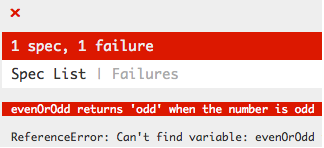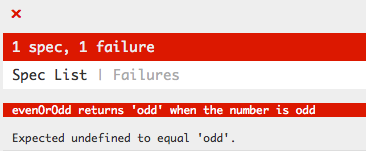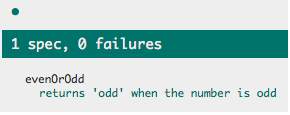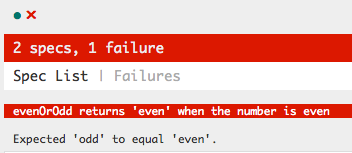# Test Your JavaScript With Jasmine

#### Posted on March 1, 2016 by By Krupal, in Javascript, Miscellaneous | 0

Testing is the best way to assure high quality of software. There are many testing tools and one is Jasmine to test our JavaScript.

What is testing?

Testing is all about verifying that your code does what it is expected to do with automated tools.

Basic example

Here created a basic evenOrodd function returning even if the value is even and odd if it is odd.

``````
var evenOrodd =function(value) {

if(value%2==0){

return'even';

} else {

return'odd';

}

};
``````

How can we verify that this code works as expected? We will take a Test-driven development (TDD) approach to this, so we will start with no code at all.

This is the content of our evenOrodd.js file right now:

``````
// nothing here, yet.
``````

First, we need a test suite. A test suite for Jasmine starts with a call to the describe function which takes two parameters: a description (string) and a function.

evenOroddSpec.js content:

``````
describe("evenOrodd",function() {

});
``````

This is our test suite for evenOrodd.

The second parameter will include our tests. Let’s start with our first test. A test is made of a call to the it function, taking two parameters: a description (string) and a function. Inside the function, we add an expectation. Let’s see how that looks like:

``````
describe("evenOrodd",function() {

it("returns 'odd' when the number is odd",function(){

expect(evenOrodd(1)).toEqual('odd');

});

});
``````

The previous example is calling our evenOrodd function with “1” as the parameter and Jasmine is expecting the return value to be “odd”.

Running the tests give us this result right now:We are getting this because evenOrodd is not even defined, let’s do this:

``````
var evenOrodd =function(){

};
``````

What would happen if we were to run the tests again?We had a different result, slowly bringing us closer to what we want.

In the TDD world, there is a thing we call “red green refactor” which means the first step is write a test that fails due to the not behaving as expected (or not being present). The green part, is writing the minimum to make that test pass. Finally, the refactor part, is making the appropriate change to have nice and clean code doing what it should be doing.

We did the “red” part of it, let’s make it pass:

``````
var evenOrodd =function(){

return'odd';

};
``````

This test will pass:Well, that’s cool, but it’s not exactly the end result we want, so let’s expand our test suite

``````
describe("evenOrodd",function() {

it("returns 'odd' when the number is odd",function(){

expect(evenOrodd(1)).toEqual('odd');

});

it("returns 'even' when the number is even",function(){

expect(evenOrodd(2)).toEqual('even');

});

});
``````

Running our tests will now have one failure, and one success:Well, that is expected as our code is not appropriate, so let’s fix it:

``````
var evenOrodd =function(value) {

if(value%2==0){

return'even';

} else {

return'odd';

}

};
``````

Now our code works as we want, it is covered by some tests. Obviously, this is very basic and non-realitisc code, but that gives you a rough idea.Best Open Source Business Intelligence Software Helical Insight is Here